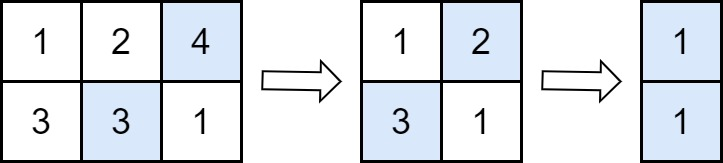2500. Delete Greatest Value in Each Row
Easy
393
23

You are given an `m x n` matrix `grid` consisting of positive integers.

Perform the following operation until `grid` becomes empty:

• Delete the element with the greatest value from each row. If multiple such elements exist, delete any of them.

Note that the number of columns decreases by one after each operation.

Return the answer after performing the operations described above.

Example 1:```Input: grid = [[1,2,4],[3,3,1]]
Output: 8
Explanation: The diagram above shows the removed values in each step.
- In the first operation, we remove 4 from the first row and 3 from the second row (notice that, there are two cells with value 3 and we can remove any of them). We add 4 to the answer.
- In the second operation, we remove 2 from the first row and 3 from the second row. We add 3 to the answer.
- In the third operation, we remove 1 from the first row and 1 from the second row. We add 1 to the answer.
The final answer = 4 + 3 + 1 = 8.
```

Example 2:```Input: grid = []
Output: 10
Explanation: The diagram above shows the removed values in each step.
- In the first operation, we remove 10 from the first row. We add 10 to the answer.
```

Constraints:

• `m == grid.length`
• `n == grid[i].length`
• `1 <= m, n <= 50`
• `1 <= grid[i][j] <= 100`
Accepted
33.9K
Submissions
42.4K
Acceptance Rate
80.1%

Seen this question in a real interview before?
1/4
Yes
No

Discussion (0)

Related Topics